# 数据结构系列，二叉平衡树的构建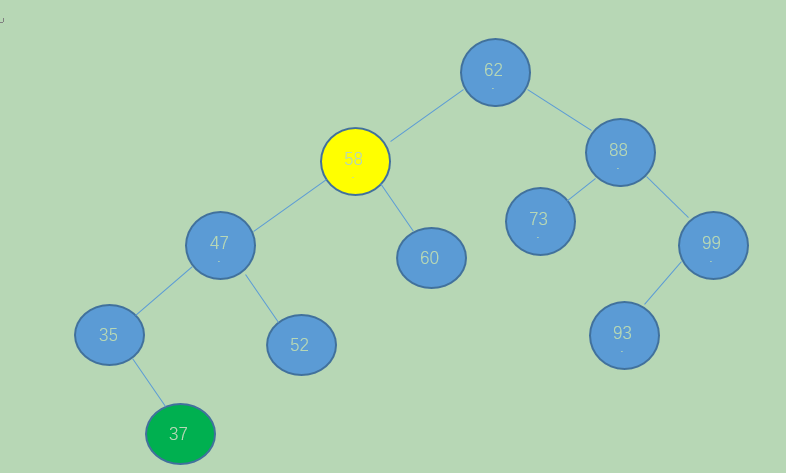Int a = {3, 2, 1, 4, 5, 6, 7, 10, 9, 8}

1. 插入前两个结点3，2时都是正常的构建，当插入第三个数1的时候，发现根结点3的BF变成了2，如图1，此时整棵树就是最小不平衡子树，因为BF为正，将其右旋，如图2。继续插入结点4。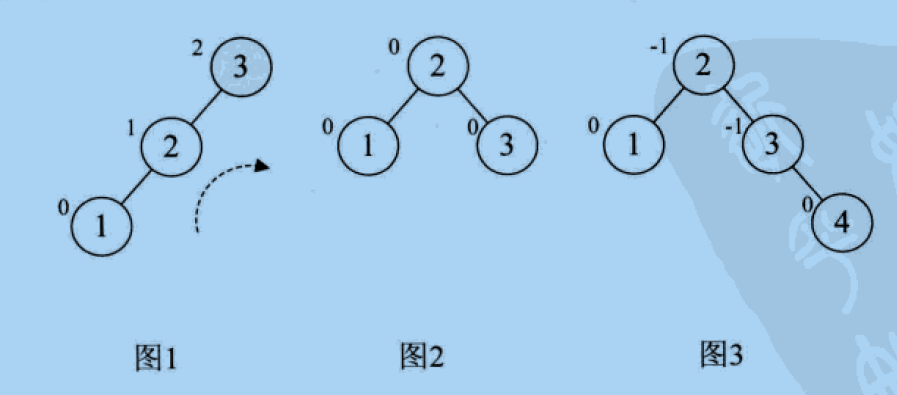2. 增加结点5时，结点3的BF变为-2，如图4，说明要左旋了，如图5.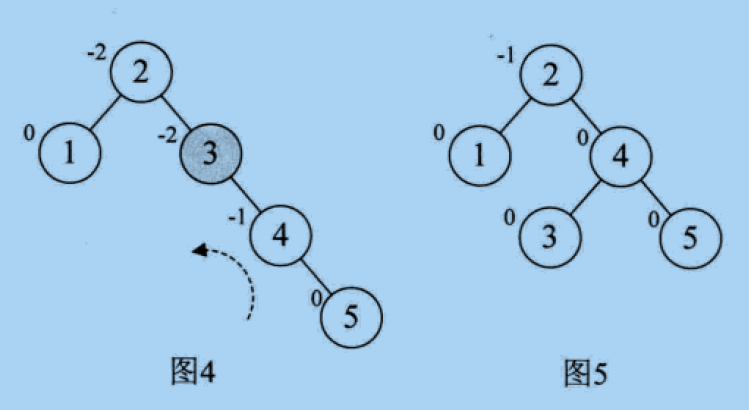3. 增加结点6时，结点2的BF变为-2，如图6，所以要左旋，如图7，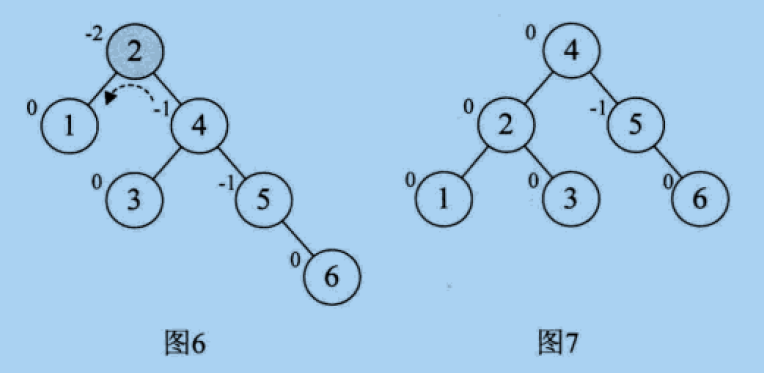4. 增加结点7时，也要左旋，如图8，图9.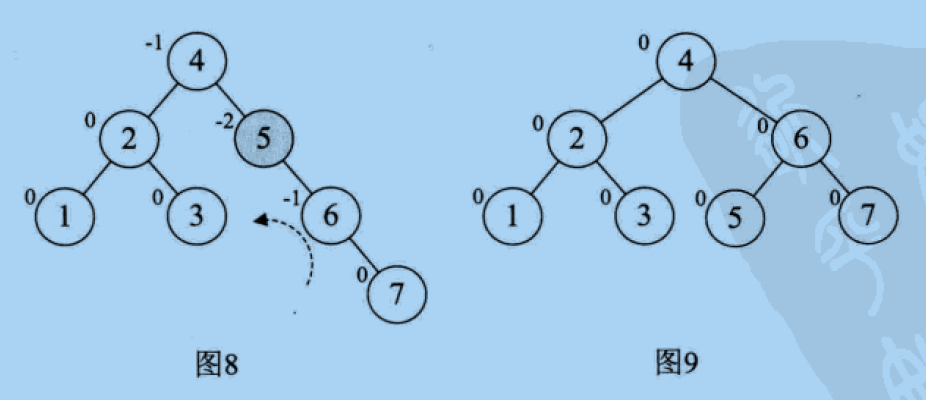5，增加结点10，没有变化，在增加结点9时，结点7的BF变为-2，如图11，如果直接左旋7,9,10，因为结点9变成10的右孩子，不符合二叉排序树的特性，所以不能简单的左旋，前面我们说，如果最小不平衡子树的BF跟他的子树的BF符号不一致，要先选择其子树，使他们的BF符号统一，在反向旋转最小不平衡子树。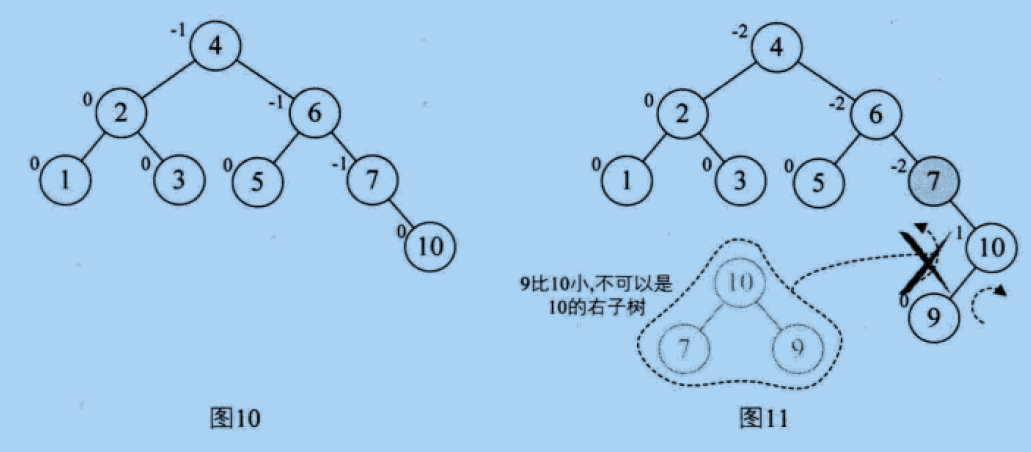所以先要对9,10做右旋，在对7，9,10做左旋，如图12，图13，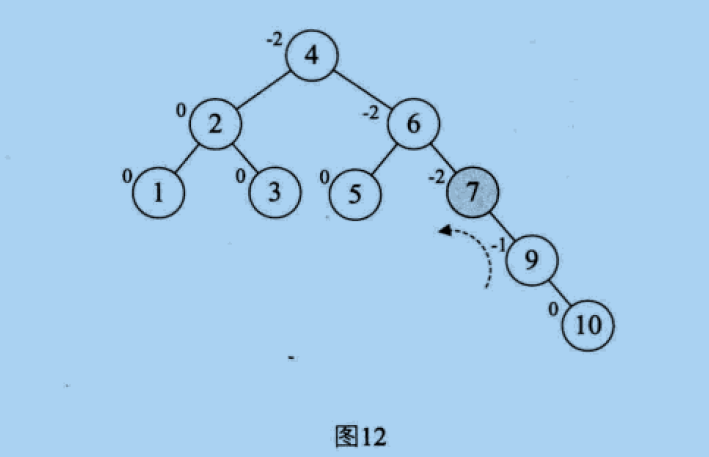6，在插入结点8，因为6的BF变为-2，它的右孩子9的BF变为1，如图14，所以要先对9右旋，如图15，在对6左旋，如图16.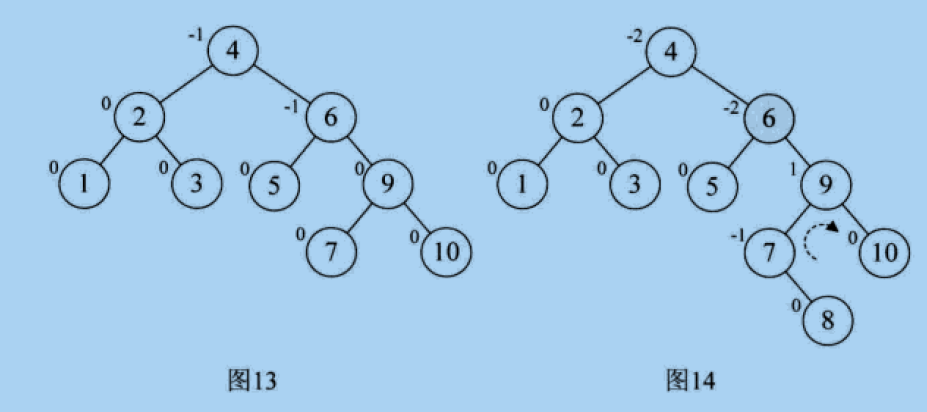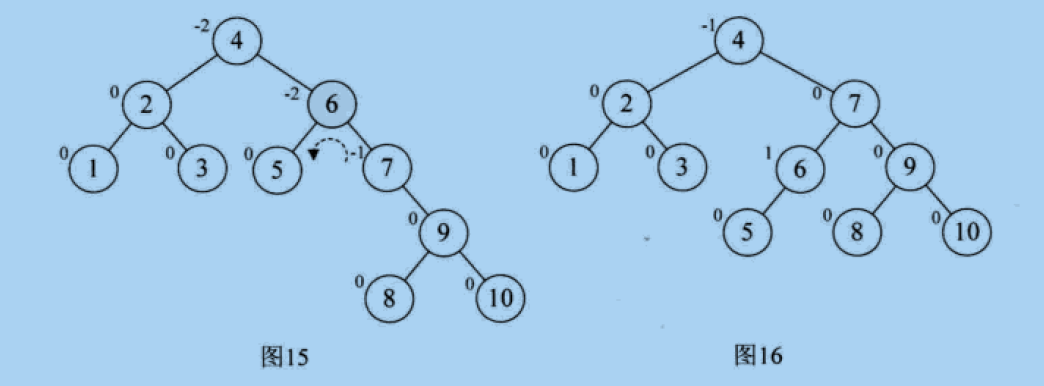代码实现：

#ifndef DATA_STRUCTURE_BINARY_BF_TREE_CLASS_H
#define DATA_STRUCTURE_BINARY_BF_TREE_CLASS_H

#define OK 1
#define ERROR 0
#define TRUE 1
#define FALSE 0
#define MAXSIZE 100 //存储空间初始分配量
#define MAX_TREE_SIZE 100 //二叉树最大结点树

typedef int Status; //表示函数结果的状态码
typedef int TreeElemType; //树节点的数据类型，暂定int
typedef TreeElemType SqBiTree[MAX_TREE_SIZE]; //顺序存储结构数组

typedef struct BiTNode {
int data;//结点的值
int bf;//平衡因子
struct BiTNode *lchild, *rchild;//左右孩子指针
}BiTNode, *BiTree;

TreeElemType Nil = 0; //表示空元素

Status Delete(BiTree *p);
void R_Rotate(BiTree *p);
void L_Rotate(BiTree *p);

#endif
#include "BinaryBFTree.h"

#include "iostream"
#include "cstdlib"
#include "cmath"
using namespace std;

#define arrayLength(array) sizeof(array) / sizeof(array)
const int array={3,2,1,4,5,6,7,10,9,8};

Status visit(BiTree T) {
if (NULL != T) {
cout << "value=" << T->data << endl;
} else {
cout << "T is null!" <<endl;
}
return OK;
}

void InOrderTraverseBST(BiTree T) {
if (NULL == T) {
//cout << "T is null!" <<endl;
return;
} else {
InOrderTraverseBST(T->lchild);
visit(T);
InOrderTraverseBST(T->rchild);
}
}

/*
*对以P为跟结点的二叉排序树做右旋，旋转后p为新的根结点。
*/
void R_Rotate(BiTree *p) {
BiTree L;
L = (*p)->lchild; //p的左孩子，为新的根结点
(*p)->lchild = L->rchild; //p的左孩子的右孩子，为p的左孩子
L->rchild = (*p);//新的根结点右孩子，为原来的p
*p = L;//指向新的根结点，原根结点的左孩子的左孩子指向没有变。
}

/*
*对p为根的二叉排序树做左旋处理，旋转后p为新的根结点
*/
void L_Rotate(BiTree *p) {
BiTree R;
R= (*p)->rchild;
(*p)->rchild = R->lchild;
R->lchild = (*p);
*p = R;
}

//最小不平衡子树的子树的高度值。
#define LH +1 //左高
#define EH 0 //等高
#define RH -1 //右高

/*
* 对T为根结点的最小不平衡子树做左平衡旋转
*/
void LeftBalance(BiTree *T) {
BiTree L, Lr;
L = (*T)->lchild;
switch(L->bf) {
//新插入结点在T的左孩子的左子树上，做右旋
case LH:
(*T)->bf = L->bf = EH;
R_Rotate(T);
break;
//新插入结点在T的左孩子的右子树上，做双旋
case RH:
Lr = L->rchild;
switch(Lr->bf) {
case LH:
(*T)->bf = RH;
L->bf = EH;
break;
case EH:
(*T)->bf = L->bf = EH;
break;
case RH:
(*T)->bf = EH;
L->bf = LH;
break;
}
Lr->bf = EH;
//对T的左子树做左旋
L_Rotate(&((*T)->lchild));
//对T做右旋
R_Rotate(T);
}
}

/*
*对T为根结点的最小不平衡子树作右平衡旋转处理，
*/
void RightBalance(BiTree *T) {
BiTree R, Rl;
R = ( * T)->rchild; /*	R指向T的右子树根结点 */
switch (R -> bf) { /*  检查T的右子树的平衡度，并作相应平衡处理 */
case RH: /*  新结点插入在T的右孩子的右子树上，要作单左旋处理 */
( * T)->bf = R -> bf = EH;
L_Rotate(T);
break;
case LH: /*  新结点插入在T的右孩子的左子树上，要作双旋处理 */
Rl = R -> lchild; /*  Rl指向T的右孩子的左子树根 */
switch (Rl -> bf) { /*	修改T及其右孩子的平衡因子 */
case RH:
( * T)->bf = LH;
R -> bf = EH;
break;
case EH:
( * T)->bf = R -> bf = EH;
break;
case LH:
( * T)->bf = EH;
R -> bf = RH;
break;
}
Rl -> bf = EH;
R_Rotate( & ( * T)->rchild); /*  对T的右子树作右旋平衡处理 */
L_Rotate(T); /*  对T作左旋平衡处理 */
}
}

/*	若在平衡的二叉排序树T中不存在和e有相同关键字的结点，则插入一个
*  数据元素为e的新结点，并返回1，否则返回0。若因插入而使二叉排序树
*  失去平衡，则作平衡旋转处理，布尔变量taller反映T长高与否
*/
Status InsertAVL(BiTree *T, int e, Status *taller) {
if (! * T) { /*  插入新结点，树“长高”，置taller为TRUE */
*T = new BiTNode;
( * T)->data = e;
( * T)->lchild = ( * T)->rchild = NULL;
( * T)->bf = EH;
*taller = TRUE;
} else {
if (e == ( * T)->data) { /*	树中已存在和e有相同关键字的结点则不再插入 */
*taller = FALSE;
return FALSE;
}
if (e < ( * T)->data) { /*	应继续在T的左子树中进行搜索 */
if (!InsertAVL( & ( * T)->lchild, e, taller)) /*	未插入 */
return FALSE;
if (*taller) { /*	已插入到T的左子树中且左子树“长高” */
switch (( * T)->bf) {/*  检查T的平衡度 */
case LH: /*  原本左子树比右子树高，需要作左平衡处理 */
LeftBalance(T); *taller = FALSE;
break;
case EH: /*  原本左、右子树等高，现因左子树增高而使树增高 */
( * T)->bf = LH; *taller = TRUE;
break;
case RH: /*  原本右子树比左子树高，现左、右子树等高 */
( * T)->bf = EH; *taller = FALSE;
break;
}
}
}
else { /*	应继续在T的右子树中进行搜索 */
if (!InsertAVL( & ( * T)->rchild, e, taller)) /*	未插入 */
return FALSE;
if (*taller) { /*  已插入到T的右子树且右子树“长高” */
switch (( * T)->bf) {/*  检查T的平衡度 */
case LH: /*  原本左子树比右子树高，现左、右子树等高 */
( * T)->bf = EH; *taller = FALSE;
break;
case EH: /*  原本左、右子树等高，现因右子树增高而使树增高  */
( * T)->bf = RH; *taller = TRUE;
break;
case RH: /*  原本右子树比左子树高，需要作右平衡处理 */
RightBalance(T); *taller = FALSE;
break;
}
}
}
}
return TRUE;
}

int main() {
BiTree T = NULL;
Status taller;
for(int i=0;i<arrayLength(array);i++) {
InsertAVL(&T,array[i],&taller);
}
InOrderTraverseBST(T);
}


/*output*/

/BinaryTree$g++ -g BinaryBFTree.cpp -o BFTree /BinaryTree$ ./BFTree
value=1
value=2
value=3
value=4
value=5
value=6
value=7
value=8
value=9
value=10


对图16按中序遍历的输出结果，是一致的。©️2019 CSDN 皮肤主题: 精致技术 设计师: CSDN官方博客